## Archive for February, 2005

24
Feb
05

### Circular Orbit TrapThis animation shows a Circular Orbit Trap transforming into a biomorph.
```(* runtime: 12 seconds *) OrbitTrap[zc_] := Module[{z = 0, i = 0, x}, While[i < 10 && Abs[z] < 2 && (i < 2 || Abs[z] > 0.3), z = z^2 + zc; i++]; x = Abs[z]^2/0.3^2; Hue[Arg[z]/(2Pi), Min[1, 2 x], Max[0, Min[1, 2(1 - x)]]]]; Show[Graphics[RasterArray[Table[OrbitTrap[xc + I yc], {yc, -1.5, 1.5, 3/274}, {xc, -2.0, 1.0, 3/274}]], AspectRatio -> 1]];```

24
Feb
05

### Mandelbrot Set Pickover Stalks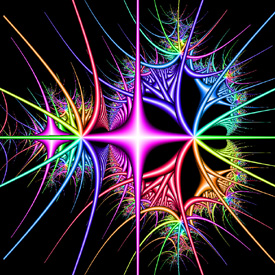Pickover stalks are cross-shaped orbit traps invented by Clifford Pickover. See also my 3D Pickover stalks fractals. Here is some Mathematica code:
```(* runtime: 33 seconds *) f[z_] := Min[Abs[{Re[z], Im[z]}]]/0.03; OrbitTrap[zc_] := Module[{z = 0, i = 0}, While[i < 100 && Abs[z] < 100 && (i < 2 || f[z] > 1), z = z^2 + zc; i++]; Hue[Arg[z]/(2Pi), Min[1, 2 f[z]], Max[0, Min[1, 2(1 - f[z])]]]]; Show[Graphics[RasterArray[Table[OrbitTrap[xc + I yc], {yc, -1.5, 1.5, 3/274}, {xc, -2.0, 1.0, 3/274}]], AspectRatio -> 1]]```

18
Feb
05

### Cubic Julia Set Fractal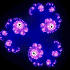zn+1 = zn3+zc, zc = -0.5-0.05i
This was one of my older favorites.

original version: Java, 6/9/01;
animated version: Mathematica 4.2, 2/18/05

```(* runtime: 10 seconds *) Julia = Compile[{{z, _Complex}}, Length[FixedPointList[#^3 + zc &, z, 100, SameTest -> (Abs[#] > 2 &)]]]; zc = -0.5 - 0.05 I; DensityPlot[Julia[x + I y], {x, -1.15, 1.15}, {y, -1.15, 1.15}, PlotPoints -> 275, Mesh -> False, Frame -> False, ColorFunction -> (RGBColor[Min[2#^2, 1], Max[2# - 1, 0], Min[2#, 1]] &)];```

This is how you can make this fractal in POV-Ray:
```// runtime: 0.5 second camera{orthographic location <0,0,-1.15> look_at 0 angle 90} plane{z,0 pigment{julia <-0.5,-0.05>,30 exponent 3 color_map{[0 rgb 0][1/3 rgb <0,0,1>][2/3 rgb <1,0,1>][1 rgb 1]}} finish{ambient 1}}```

16
Feb
05

### Mandelbrot Set Zoom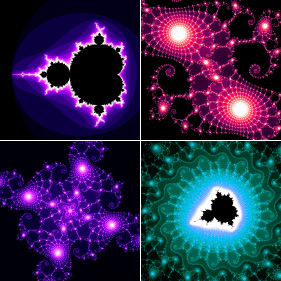zn+1 = zn2+zc
This animation zooms in on the Mandelbrot set by a factor of 1015. At this high resolution, double precision numbers are inadequate. Therefore, this animation was created using “double-double” precision numbers, adapted from Keith Brigg’s double-doubles. See also my Java program and C program.

Mandelbrot Set Zoom – original version: Java, 5/24/01; animated version: C++, 2/16/05 Here is some Mathematica code for this fractal:
```(* runtime: 1 minute *) Mandelbrot[zc_] := Module[{z = 0, i = 0}, While[i < 100 && Abs[z] < 2, z = z^2 + zc; i++]; i]; DensityPlot[Mandelbrot[xc + I yc], {xc, -2, 1}, {yc, -1.5, 1.5}, PlotPoints -> 275, Mesh -> False, Frame -> False, ColorFunction -> (If[# != 1, Hue[#], Hue[0, 0, 0]] &)];```

Here is a faster version:
```(* runtime: 7 seconds *) Mandelbrot = Compile[{{zc, _Complex}}, Length[FixedPointList[#^2 + zc &, zc, 100, SameTest -> (Abs[#] > 2 &)]]]; DensityPlot[Mandelbrot[xc + I yc], {xc, -2, 1}, {yc, -1.5, 1.5}, PlotPoints -> 275, Mesh -> False, Frame -> False, ColorFunction -> (If[# != 1, Hue[#], Hue[0, 0, 0]] &)];```

POV-Ray has a built-in function for this fractal:
```// runtime: 0.5 second camera{orthographic location <-0.5,0,-1.5> look_at <-0.5,0,0> angle 90} plane{z,0 pigment{mandel 100 color_map{[0 rgb 0][1/6 rgb <1,0,1>][1/3 rgb 1][1 rgb 1][1 rgb 0]}} finish{ambient 1}}```

12
Feb
05

### Frequency Filtered Random Noise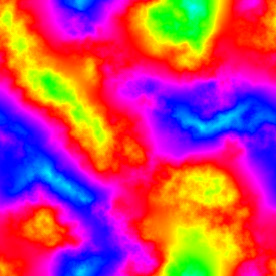Frequency Filtered Random Noise : another variation of frequency filtered random noise.
```(* runtime: 20 seconds *) n = 275; SeedRandom; fourier = Fourier[Table[Random[], {n}, {n}]]; filter = Table[fourier[[i, j]]/((j/n - 0.5)^2 + (i/n - 0.5)^2), {i, 1, n}, {j, 1, n}]; ListDensityPlot[Map[Abs, InverseFourier[filter], {2}], Mesh -> False, Frame -> False, ColorFunction -> (Hue[# + 0.5] &)];```

10
Feb
05

### Diffusion Limited Aggregation (DLA) Fractal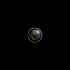This fractal simulates a diffusive growth process similar to that often found in nature. It is generated by single points that randomly drift around until they find something to stick to. The title on this page was generated using this technique.

Diffusion Limited Aggregation (DLA) FractalMathematica 4.2, 8/12/04; C++ version: 2/10/05

```(* runtime: 1 minute *) n = 100; ix = iy = n/2; i = 0; SeedRandom; image = Table[0, {n}, {n}]; Do[{ix, iy} = Floor[n(0.5 + 0.1{Cos[theta], Sin[theta]})] + 1; image[[ix, iy]] =1, {theta, 0, 2 Pi, Pi/180}]; While[(ix - n/2)^2 + (iy - n/2)^2 < (n/2)^2, theta = 2 Pi Random[]; {ix, iy} =Floor[n(1 + {Cos[theta], Sin[theta]})/2]; drift =True; While[drift, {ix, iy} = Mod[{ix + Random[Integer, {-1, 1}], iy + Random[Integer, {-1, 1}]}, n]; drift = Plus @@ Flatten[image[[Mod[ix + {-1, 0, 1}, n] + 1, Mod[iy + {-1, 0, 1}, n] + 1]]] == 0]; image[[ix + 1, iy + 1]] = 1 - i/n^1.5; i++]; ListDensityPlot[image, Mesh -> False, Frame -> False];```

05
Feb
05

### Gravitational Lensing of a Black HoleGravitational Lensing of a Black Hole : According to Einstein’s Theory of Relativity, the intense gravity of a black hole can bend light into a circle called an Einstein Ring. See also Event horizon, Schwarzschild radius, photon sphere. The following code is only an approximation:
```(* runtime: 30 seconds *) Clear[stars]; SeedRandom; stars[{x_, y_}] = Sum[Exp[-500((x - Random[])^2 + (y - Random[])^2)/Random[]^2], {10}]; DensityPlot[Module[{r = x^2 + y^2}, If[r 275, PlotRange -> {0, 1}, Mesh -> False, Frame -> False]```

## Welcome !

You will find here some of my favorite hobbies and interests, especially science and art.

I hope you enjoy it!

Subscribe to theRSS feed to stay informed when I publish something new here.

I would love to hear from you! Please feel free to send me an email : bugman123-at-gmail-dot-com

## TopicsBerna Blalack on Magnetic Pendulum Strange…Daan on Magnetic Pendulum Strange…Sebastian Schepis on Diffusion Limited Aggregation…mohammad_andito on CFM56-5 Turbofan Jet Engi…SasQ on Magnetic Field of a Solen… OUPblog » Blog… on Diamond Light Dispersion Complex Roots on Polynomial Roots Joukowski airfoils |… on Joukowski AirfoilKarim Alame on Flapping WingREJISH J on Joukowski Airfoil SOLINOID | Materials… on Magnetic Field of a Solen…Emanuele on 4D “Squarry” Julia…Emanuele on Hydrogen Electron Orbital Prob…Tim on Mandelbrot Set Pickover S…khankasi1 on 4D Quaternion Mandelbrot …